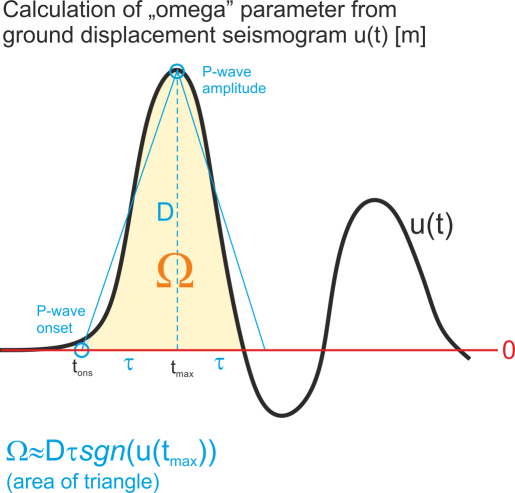# focimt

## What is the magnitude limit for application of fociMT or hybridMT algorithms?

The fociMT algorithm is point-source moment tensor inversion and the fault rupture processes (e.g. directivity or spatial changes in elementary moment tensors) are NOT considered. As such, the algorithm is valid for events with simple source time functions that can be represented by a single pulse. Therefore, it is suggested to use the algorithm for events with moment magnitudes not exceeding Mw 4.

## Is fociMT algorithm based on full waveform inversion?

No. The input for moment tensor inversion using fociMT algorithm is area below first P-wave ground displacement pulse with sign information. As such, the inversion algorithm fall into the class of “amplitude MT inversion” algorithms (i.e. it is different from e.g. ISOLA algorithm)

## What is the “omega” parameter in the focimt input file and how to calculate it?“omega” parameter which is a part of ASCII input file is basically the area below the first P-wave ground displacement pulse (in time domain) or equivalently the spectral level taken from the amplitude spectrum of ground displacement pulse. The unit of “omega” parameter is [m * s] (meter times second), and it comes from the fact that we multiply the amplitude of first P-wave pulse using ground displacement seismogram in [m] by the half of duration of the first P-wave pulse in [s]. Regardless of whether time or frequency domain was used to extract the “omega” value, the parameter should contain the sign information. The sign of “omega” is in accordance with the seismological convention, i.e. it is positive for the positive first P-wave ground displacement amplitude (i.e. ground motion AWAY from the seismic source) and negative otherwise (ground motion TOWARDS the seismic source).

In case of simple impulsive source time functions it is typically not necessary to calculate the integral of ground displacement first P-wave pulse. Instead, the amplitude D (cf. picture) including sign information of first P-wave pulse may be taken from ground displacement seismogram (the seismogram should be calibrated to [m]) and multiplied by the the rise time (i.e. time interval between the P-wave onset and P-wave amplitude, t_max-t_ons in seconds [s]). In this case, the integral of the pulse is simply replaced by the area of the triangle.

## What full moment tensor decomposition scheme is used in focimt.m?

The MATLAB wrapper focimt.m has no option for changing the decomposition scheme, which is currently fixed to Jost and Herrmann’s (1989) approach. However, the focimt application has implemented an alternative decomposition scheme based on Vavrycuk’s (2001) approach. In focimt application this is specified by option -d D or -d Y (see the documentation of focimt application for details). Therefore, the hardcoded decomposition scheme in focimt.m may be changed from Jost and Herrmann’s to Vavrycuk’s approach simply by changing the following line from (note change from “D” to “Y” in option -d):

`commandline = ['-i ' temp '.txt -d DWAFTUMVE -o ' temp ' -s ' solutions ' -n ' normfunc ' ' bbsize ' ' bbformat ' ' bbproj ' ' jacknife ' ' bootstrap ' ' vmodel ' ' ball];`

to

`commandline = ['-i ' temp '.txt -d YWAFTUMVE -o ' temp ' -s ' solutions ' -n ' normfunc ' ' bbsize ' ' bbformat ' ' bbproj ' ' jacknife ' ' bootstrap ' ' vmodel ' ' ball];`

Future version of focimt.m implement the decomposition switch parameter.